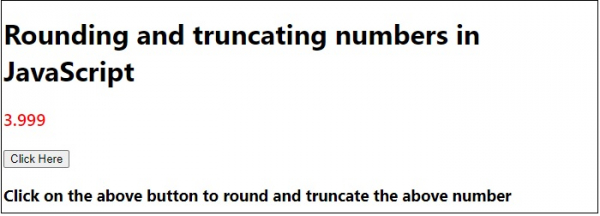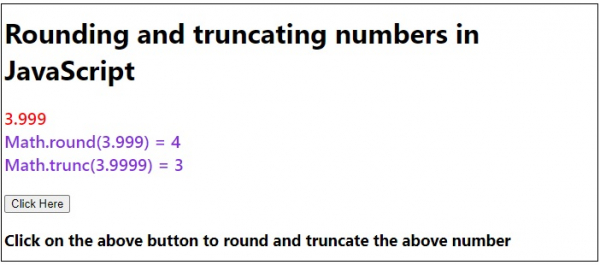# Rounding and truncating numbers in JavaScript.

There are two functions in JavaScript for rounding and truncating numbers: Math.round() and Math.trunc() respectively −

• Math.round() − = It rounds the decimal number to the nearest integer value.
• Math.trunc() − = It simply removes the fractional part of the decimal number and converts it to a whole number.

Following is the code for rounding and truncating numbers in JavaScript −

## Example

Live Demo

<!DOCTYPE html>
<html lang="en">
<meta charset="UTF-8" />
<meta name="viewport" content="width=device-width, initial-scale=1.0" />
<title>Document</title>
<style>
body {
font-family: "Segoe UI", Tahoma, Geneva, Verdana, sans-serif;
}
.result,
.sample {
font-size: 20px;
font-weight: 500;
color: blueviolet;
}
.sample {
color: red;
}
</style>
<body>
<h1>Rounding and truncating numbers in JavaScript</h1>
<div class="sample">3.999</div>
<div class="result"></div>
<br />
<h3>Click on the above button to round and truncate the above number</h3>
<script>
let resEle = document.querySelector(".result");
let BtnEle = document.querySelector(".Btn");
resEle.innerHTML = Math.round(3.9999) + "<br>";
resEle.innerHTML += Math.trunc(3.9999) + "<br>";
});
</script>
</body>
</html>

## Output